Quantity: 0

Total: 0,00

0

# Grouping of cuboids

### Grouping of cuboids

This animation demonstrates various types of cuboids through everyday objects.

Mathematics

Keywords

cuboid, square prism, cube, grouping of cuboids, set, grouping, mathematics

Related items

### Scenes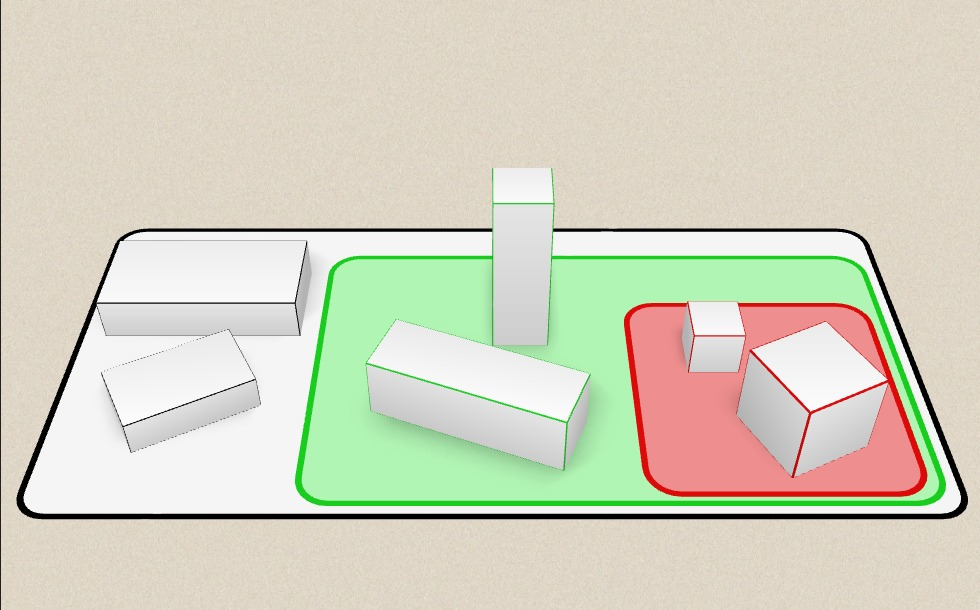### Grouping of cuboids

• cuboids
• square prisms
• cubes

One way of grouping the cuboids is according to their formation.

A cuboid is a prism with a rectangular base.

Square prisms are a special type of cuboids.

Square prisms are cuboids with a square face. The face opposite to it is also a square. If there are no other square faces, the remaining 4 faces are congruent rectangles.

Cubes are a special type of square prisms, therefore they belong to a different category.

A cube is a cuboid with six square faces. Put differently, a cube is a square prism where the height and the length of the edge of the base are equal. Or, a cube is a cuboid with at least 3 square faces. If two of these are opposite to each other, we have a square prism, so the third face is a lateral face of the prism. In other words all the faces of the resulting prism are congruent squares.

If any two of the 3 faces are not opposite to a third, they share a common vertice. Being rectangles, all their opposite faces are congruent with them, so they are also squares.

### Related items#### Cuboid

A cuboid is a polyhedron with six rectangular faces.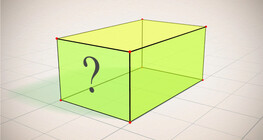#### Cuboid (exercises)

Edges, diagonals and faces of a cuboid can be identified by its vertices.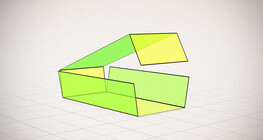#### Nets of a cuboid (exercises)

This animation demonstrates the different nets of a cuboid and includes a game.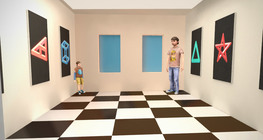#### Ames room

An Ames room is a distorted room that is used to create an optical illusion.#### Cube puzzle

Building cubes shown in several views from the available unit cubes aids spatial vision and other skills.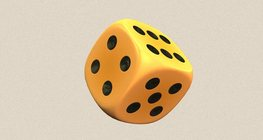#### Dice

Regular dice can be used for solving statistical and probability exercises.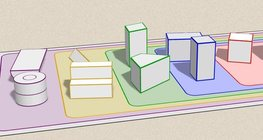#### Grouping of solids

This animation demonstrates various groups of solids through examples.#### Perimeter, area, surface area and volume

This animation presents the formulas to calculate the perimeter and area of shapes as well as the surface area and volume of solids.#### Ratio of volumes of similar solids

This 3D scene explains the correlation between the ratio of similarity and the ratio of volume of geometric solids.#### Platonic solids

This animation demonstrates the five regular three-dimensional (or Platonic) solids, the best known of which is the cube.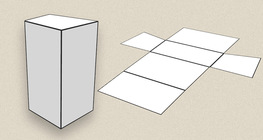#### Prisms

This animation demonstrates several types of prisms, from general to regular.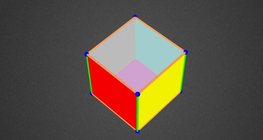#### Colouring a cube

Colouring the vertices, edges and faces of a given cube according to the criteria specified in the exercise.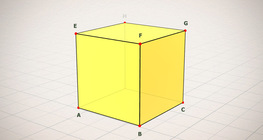#### Cube (exercises)

Edges, diagonals and faces of a cube can be identified by its vertices.#### Cube of cubes

An exercise about the regular hexahedron built from unit cubes to help deepen your knowledge of cubes.#### Net of a cube (exercises)

Not all nets consisting of 6 congruent squares are foldable into cubes.#### Volume and surface area (exercise)

An exercise about the volume and surface area of solids generated from a ´base cube´.